# Jeans instability

In the interstellar model, molecular clouds are the sites of star formation. Clouds must be in hydrostatic equilibrium for stability, which requires that internal pressure be in equilibrium with gravitational pressure or tuned to it. Instability happens when gravitational pressure exceeds internal gas pressure, which is insufficient to avert gravitational collapse. The uniform interstellar model is broken up into clumps by a fluid dynamical process called Jeans instability, which starts the instability. In the interstellar model, this instability leads to the collapse of the molecular cloud and star formation.

Let's say the gas distribution was initially consistent and there was a localized disturbance. The extra pressure in this area would cause acoustic waves to form, which would then propagate through the compressed air in the surrounding areas, bringing the gas back to its uniform state. Yet, because of the accelerated gravitational collapse, more gas is generally drawn into the compressed zone. However, the enhance evolve depends on whether the acoustic waves or enhanced gravity will win over. If the region of compression is small than acoustic wave win over. If the region of compression is large than critical size (Jeans size), then the gravity may overlaps the acoustic waves, pulling more material into the region and triggering an instability. The initial state is roughly speaking a gas cloud that begins to collapse due to its own gravitation. When a cloud's mass is great enough, its gravitational energy outweighs its kinetic energy, causing the cloud to collapse.

When the heat pressure is insufficient to balance the gravitational forces acting on the system, Jeans instability, a gravitational instability, can happen in a fluid system. The instability was first described by the physicist Sir James Jeans in 1902. The critical mass will depend on this thermodynamical parameter pressure (responsible for acoustic waves) and density (responsible for gravity). Thus the critical mass is of the form of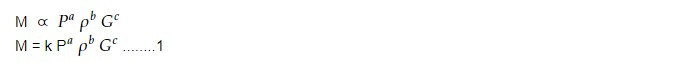where k is dimensionless constant, we can find the value of a, b and c dimensionallyEquating we get

a + b -c = 1

or, -a -3b+3c = 0

or, -2a -2c = 0

Solving we get, a =3/2, b= -2 , c= -3/2

With these values equation i becomes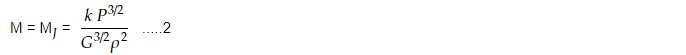This is called Jean's Mass unit. Jean's Mass is a unit of mass used in astrophysics, named after the French astrophysicist James Hopwood Jeans. It is defined as the critical mass of a cloud of interstellar gas, below which the cloud cannot collapse to form a star. The proportionally constant k depends upon the nature of perturbation. The perturbation depends on the speed of acoustic waves and adiabatic index (r) for slowly varying perturbation. The Jean's mass is an important concept in the study of star formation, as it provides a threshold for the minimum size of a cloud of gas required for it to collapse and form a star. If the mass of the cloud is less than the Jean's mass, then thermal pressure and other factors prevent it from collapsing under gravity.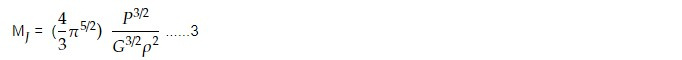Since molecular cloud is extremely cold, we can apply equation of state.

P = 𝜌KT / 𝜇mH

where K -> Maxwell Boltzmann constant

mH -> mass of hydrogen

𝜇 -> mean molecular weight

Substituting in equation 3 , we get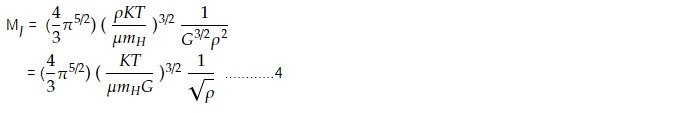The value of Jean's mass depends on the temperature, density, and composition of the gas. For typical interstellar gas conditions, the value of Jean's mass is around 0.5 solar masses, which means that a cloud of gas needs to have a mass greater than this value in order to collapse and form a star. So if we know the temperature and density of molecular cloud, we can estimate Jeans mass.

Jeans length is the critical radius of cloud where thermal energy which causes the clouds to expand is opposed by gravity. This causes clouds to collapse. The radius of the cloud can be estimated as,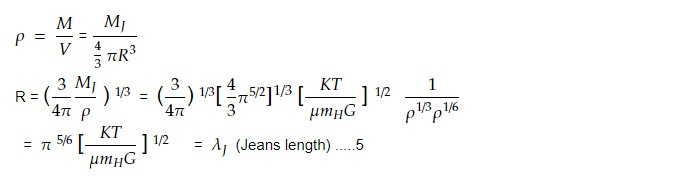Equation 5 gives Jeans length. The oscillation wavelength below which stable oscillation instead of gravitational collapse occurs is known as the Jeans length. When a cloud reaches this length, it neither collapses nor contracts. The fundamental cause of the universe's non-uniform distribution of stuff is Jeans instability. It is thought that stars and galaxies are the remnants of perturbation, which began expanding because of Jeans instability. The procedure is made considerably more difficult by the existence of magnetic field and angular momentum.

This concept is important in many areas of astrophysics, including the formation and evolution of stars, galaxies, and large-scale structures in the universe.

Jeans instability is an important concept in astrophysics, as it plays a crucial role in the formation of structures in the universe, from the first protostars in the early universe to the formation of galaxy clusters.

This note is a part of the Physics Repository.